Quantum Electrodynamics has Zero Radius of Convergence

Summarized from Toichiro Kinoshita

Quantum Electrodynamics is the best tested theory on earth (perhaps in the universe, depending on how many aliens there are). The practitioners (starting with Schwinger and Feynman, and now being led by Tom Kinoshita here at Cornell) typically calculate ``g-2'' for the electron. They've calculated it as a series to eighth order in the electron charge e, or more properly to fourth order in the fine-structure constant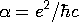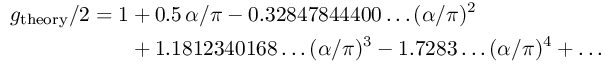These calculations aren't easy. The first term is represented by a Feynman diagram with one closed loop, and requires no more than a page or two of hand calculation. The second term represents seven diagrams, and took seven years. Seventy two Feynman diagrams are needed for the third term, and all of them have been evaluated exactly using symbolic manipulation programs on computers, after nearly thirty years of hard work. The fourth term requires the evaluation of 891 four-loop Feynman diagrams, and has been estimated numerically using large-scale computations on supercomputers.

Extremely clever experiments have measured this same number. For the electron, it is measured to be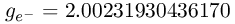and for the positron (the anti-electron) it's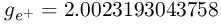(If the electron and positron did not have the same values for g, that would be an amazing surprise.)

Now, to compare experiment and theory, we need to know the value of the fine-structure constant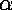. The measurements of the value of this constant aren't as accurate as those of g-2! One can measureusing the quantum Hall effect, get 1/137.0360037(27) (an accuracy of 0.020ppm), and predict

(g-2)qH =1,159,652,156.4(22.9) x 10-12
One can measureusing the ac Josephson effect to be 1/137.0359770(77) (0.056ppm), and predict
(g-2)acJ =1,159,652,378.0(65.3) x 10-12
Or, one can measure Planck's constant h and the mass of the neutron, and deriveto be 1/137.03601082(524) (0.039ppm) to predict
(g-2)h/mn =1,159,652,092.2(44.4) x 10-12
The numbers in parentheses are due to the uncertainty in the experimental value of; the errors in the computer-measurement of the theoretical formula is much smaller. If you trust the theory, you can work backwards to an even better estimate of: 1/137.035999710.

This is undoubtedly the most accurate prediction ever made, and one of the most difficult. It's also one of the most accurate measurements ever made.

What's the point? Well, it turns out that this series does not converge! Freeman Dyson gave a wonderful argument that the radius of convergence of this function is zero. He noticed that the theory was sick (unstable) for negative, (complex electron charge), no matter how small alpha was. Since any circle about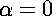includes some of the sick region, the series could not converge! Just like Stirling's formula, the theory is mighty useful even though it won't keep improving as we calculate more and more terms.

This was the inspiration for our work showing that elastic theory has zero radius of convergence.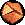Statistical Mechanics: Entropy, Order Parameters, and Complexity, now available at Oxford University Press (USA, Europe).